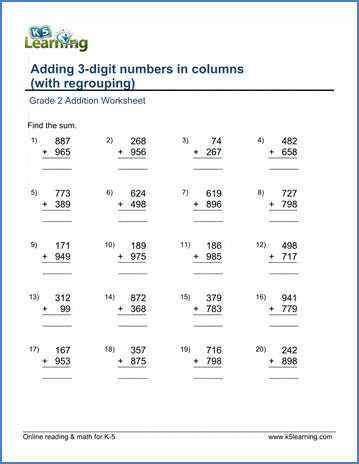## What is regrouping?

Regrouping means to arrange something into a new group or groups. Applied to addition, this means regrouping numbers into new groups.

We often use columns when adding 3-digit numbers with regrouping. It’s easier to apply the regrouping when the ones are stacked on top of each other, the tens are stacked, and the hundreds are stacked.

We now think of the ones as one group, the tens as one group, the hundreds as one group, etc.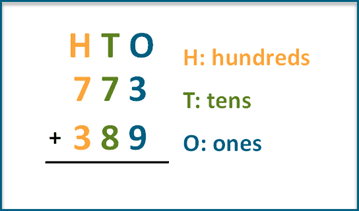## When do you use regrouping?

Regrouping is used when the sum of the values in one place value column (or group as we referred to above) is more than nine.

For example, let’s use the above example: 773 + 389. In this case, you need to regroup. Let’s take this step by step.

We start with the ones column. We add 3 to 9, which makes 12. Broken into its groups that is 2 ones and 1 ten. We, therefore, move the 1 ten to the tens column.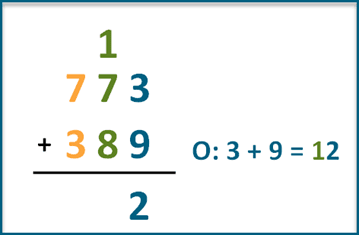Now, turn your attention to the tens. In this column we add 10 + 70 + 80 = 160. We have a number larger than 9 tens, so the 1 moves to the 100 column.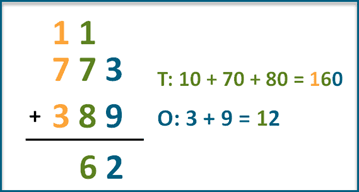Finally, we add the hundreds: 100 + 700 + 300 = 1,100. Again, we have a number larger than 9 hundreds, we need to move the 1,000 to a new thousands column.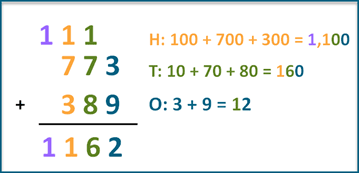The answer to 773 + 389 is 1,162.

## Adding 3-digit numbers with borrowing video

We’ve created a 60 second video to demonstrate visually how to add three-digit numbers with regrouping.